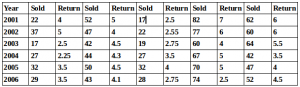## Data Interpretation For SBI PO : Set – 45

D.1-5) As part of renovation of the home, flooring, painting and carpeting of a hall is to be undertaken. The hall has length 10m, breath 12m and height 5m. The cost of flooring is Rs.2500perm2 , painting is Rs.600 per sq m and carpeting is Rs.500 per sq m

1) What will be the cost of carpeting the hall leaving aside the area of 10m *4.5m for the wooden sofa?

a) 37,500

b) 35,700

c) 35,500

d) 34,500

e) None of these

a)

500(10*12 – 10*4.5) = 500*75 = 37,500

2) What will be the cost of flooring the house if the contractor offered 10% discount on the total cost ?

a) 2,70,300

b) 3,00,000

c) 3,30,000

d) 2,70,000

e) None of these

d)

10*12*2500 = 3,00,000

3,00,000*10/100 = 30,000

3,00,000 – 30,000 = 2,70,000

3) What will be the total cost of flooring and painting the Ceiling ?

a) 3,72,000

b) 3,70,000

c) 3,27,000

d) 3,00,000

e) None of these

a)

10*12*2500 + 10*12*600 = 3,00,000 + 72,000 = 3,72,000

4) What will be the difference between the cost of painting the house on length side and the wall on the breath side provided neither of the walls has a door or a window

a) 5000

b) 4000

c) 6000

d) 6400

e) None of these

C)

12*5- 10*5  = 60 – 50 = 10

10*600 = 6000

5) If the cost of the plastering is Rs.350 then what will be the cost of the plastering the wall ?

a) 16,500

b) 17,000

c) 17,250

d) 17,500

e) None of these

d)

10*5 = 50

50*350 = 17,500

D.6-10) Study the information carefully to answer the given questions
No of 5 types of books sold and returned(due to missing of pages) by a publisher over the years(data in 10000)6) In the case of B2 type of books, in which year was the ratio of return to sold highest among the five years ?

a) 2001

b) 2002

c) 2003

d) 2004

e) 2005

c)

2001 = 5/52 =0.0962

2002 = 4/47 = 0.0851

2003 = 4.5/42 = 0.1071

2004 =4.3/44 = 0.0977

2005 = 4.5/50 = 0.09

2006 = 4.1/43 = 0.0953

7) In the year 2002 ,  ratio of book return to sold was  below 8.5% in which type of books  ?

a) B1

b) B2

c) B3

d) B4

e) B5

d)

B1 =(5*100)/37 = 13.51%

B2 =(4*100)/47 = 8.51%

B3 =(2.55*100)/22 = 11.59%

B4 =(6*100)/77 = 7.79%

B5 =(6*100)/60 = 10%

8) In the case of B5 type of books, in which year was the return to sold rate is lowest among the 6 years ?

a) 2006

b) 2002

c) 2003

d) 2005

e) 2004

e)

2001 = 6/62 = 0.0968

2002 = 6/60 = 0.10

2003 = 5.5/64 = 0.0859

2004 = 3.5/42 = 0.0833

2005 = 4/47 = 0.0851

2006 = 4.5/52 = 0.0865

9) The acceptable B5 type of books in 2003 was what percent of that of that in 2002 ?

a) 108.33%

b) 103.88%

c) 108.44%

d) 103.54%

e) None of these

a)

(64-5.5)*10,000 = 585000………….2003

(60-6)*10,000 = 540000……………..2002

% = (585000*100)/540000 = 108.33%

10) The number of B3 type of books returned in 2002 is approximately what % more or less than B4 type of books rejected in 2003 ?

a) 36.2%

b) 32.65%

c) 36.25%

d) 15.65%

e) None of these